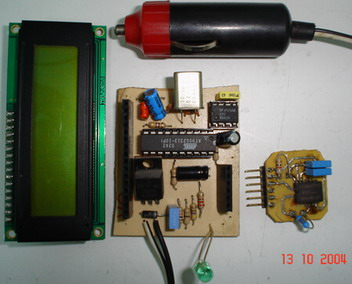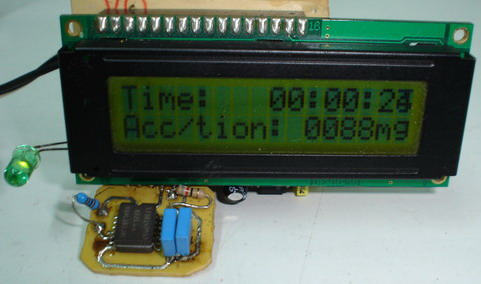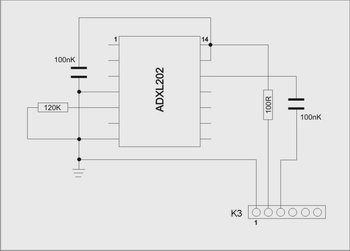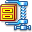Introduction

The circuit is drawn for measurement of acceleration from  –1000 mg until + 1000 mg. It can be placed in kantra'n the car and be supplied from the sheath of electric lighter. The circuit includes one indicative LED and a screen LCD.The shematic diagram of the circuit.The connections for ADXL202.

Description

The measurement of acceleration becomes with the use of IC from Analog Devices, the ADXL202. The particular element contains 2 autonomous vertical between them sensors, for the measurement in two axes. For the particular application becomes use of only it's one. Acceleration meter measures dynamic or static acceleration from  –2g until 2g (where g is the acceleration of gravity) and when this is equal to zero, exit is a signal PWM, with Duty Cycle 50%. As long as the acceleration increases, increases also the Duty Cycle, with biggest response the 75% (in the 2g), while for acceleration  –2g, the Duty Cycle is 25%. As conclusion from more, has that for change 1g, is altered also the Duty Cycle of exit at 12,5%.

The measurement of Duty Cycle of response of acceleration meter becomes with the help of Timer1 (16 bit). The period of signal of expense, has been fixed in the 1,12 msec. The frequency of oscillation of microcontroller is in the 3,6864 MHz and this means that it's each circle of access lasts 0,27 * 10 -6  sec. Using timer1, in frequency of measurement ck/1, the biggest feasible counted time is the 65536 * 0.27 * 10 -3  msec = 17.778 msec, which is bigger than period (1,12 msec). So, any price Duty Cycle it is possible to be measured.

Before the beginning of measurements, it precedes a process of accelerator’s initializing. At this process it is doing 16 measurements, and then is found their mean. In short time interval, it is considered that the acceleration was not altered also thus the result, us gives the point of null acceleration. In other words at this process becomes approach of value Duty Cycle, that corresponds in null acceleration. From now on, after each measurement, it will be removed from Duty Cycle, the null acceleration.

At the placement of appliance, the completed ADXL202 should be always parallel with the surface of ground and the pins 1 and 14 they see front part of car.  The LCD is connected at the K2 connector and the ADXL202 is connected at the K1 connector (K3 is connected with K1).

Function

With the shooting of switch of starter, the circuit is supplied. In the beginning, and as long as LED is power-off, becomes initializing of meter. Acceleration meter is will be always placed so as to it measures the acceleration at the address of movement of vehicle. As long as however well and it is placed this, will always exist a component vertical in the movement and with time to the centre of ground. This component is the acceleration of gravity and which is always removed from each measurement. As soon as the LED turns on (in minimal time), then the initializing it has become. From now on the meter measures the acceleration and him presents in the screen. When is presented the symbol "-", it means that it is deceleration.

In the above department of screen is presented the clue of total time interval, that your car is in operation. The operation of this clock is based on vibrations that come in the pin External Interrupt 0, from exterior circuit based in the timer LM555.

In the future it is is published new circuit, with the himself characteristically, that will use the second exit acceleration meter, for the measurement of centripetal acceleration, at the movement of car.Designed by: Michael Karaoglanidis

Published at 06.10.2004 by Serasidis Vasilis Http://www.serasidis.gr